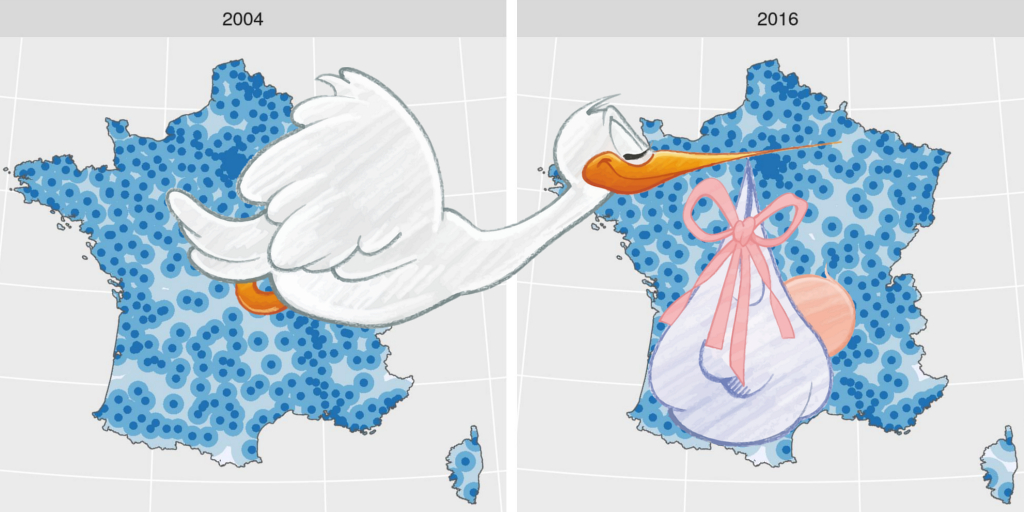# Introduction to mapping with {sf} & Co.

At the “Rencontres R 2018” in Rennes, I proposed a brief introduction to mapping using the recent {sf} package and some other interesting packages. This blog post is an extended version of my presentation allowing me to share the code of the different maps that appeared on it. It’s still a brief introduction.

The original “RR2018” presentation in French is on my github repository

R-code is folded in this blog post, clic on the “CODE” button to unfold it

## Mapping in R

All classical operations on spatialized data can be completely performed in :

• Reading and exploration of spatialized / geographic data
• Attributes manipulation (creation, selection)
• Geomatics processing (intersection, joint, surface calculation)
• Map creation (static, interactive)

Packages

``````library(dplyr)
library(sf)
library(ggplot2)
library(tmap)
library(leaflet)``````

## Projections: the ❤️ of the problem

A map is a 2-dimensional representation of all or part of our planet which, sorry to tell you this, is a sphere!Images of my previous article “spatial interpolation on earth as a 3D sphere”

To create a 2-dimensional map, a projection must be made. The areas you study will be more or less distorted by the projection you chose. One day or another (and it will happen faster than you think!), you will have a problem with a projection. If you don’t know what a projection is, you won’t find the source of your problem! So, if you want to do mapping with R without going through training (such as training at ThinkR for example), you will have to learn and understand what a projection is.

=> Remember your first function `sf::st_transform(x, crs)` to change coordinate system

### Projection of Metropolitan France

I’m not gonna give you a training on projections here. That would be very helpful, but that is not the core of this article. I just present you the map of Metropolitan France that you can download on my github, although these data are originally from the ign website.

``````# extraWD <- "."
if (!file.exists(file.path(extraWD, "departement.zip"))) {
githubURL <- "https://github.com/statnmap/blog_tips/raw/master/2018-07-14-introduction-to-mapping-with-sf-and-co/data/departement.zip"
unzip(file.path(extraWD, "departement.zip"), exdir = extraWD)
}
departements_L93 <- st_read(dsn = extraWD, layer = "DEPARTEMENT",
quiet = TRUE) %>%
st_transform(2154)``````
• 🌍 Geographical coordinates
• In degrees, minutes
• EPSG: 4326
• Used to share data
``````ggplot(departements_L93) +
aes(fill = CODE_REG) +
scale_fill_viridis_d() +
geom_sf() +
coord_sf(crs = 4326) +
guides(fill = FALSE) +
ggtitle("Coord. géographiques") +
theme(title = element_text(size = 16),
plot.margin = unit(c(0,0.1,0,0.25), "inches"))``````• 🗺 Projection in Lambert 93
• Coordinates in meters
• EPSG: 2154
• A projection to show Metropolitan France on a flat map
``````g_dept <- ggplot(departements_L93) +
aes(fill = CODE_REG) +
scale_fill_viridis_d() +
geom_sf() +
coord_sf(crs = 2154, datum = sf::st_crs(2154)) +
guides(fill = FALSE) +
ggtitle("Lambert 93") +
theme(title = element_text(size = 16))
g_dept``````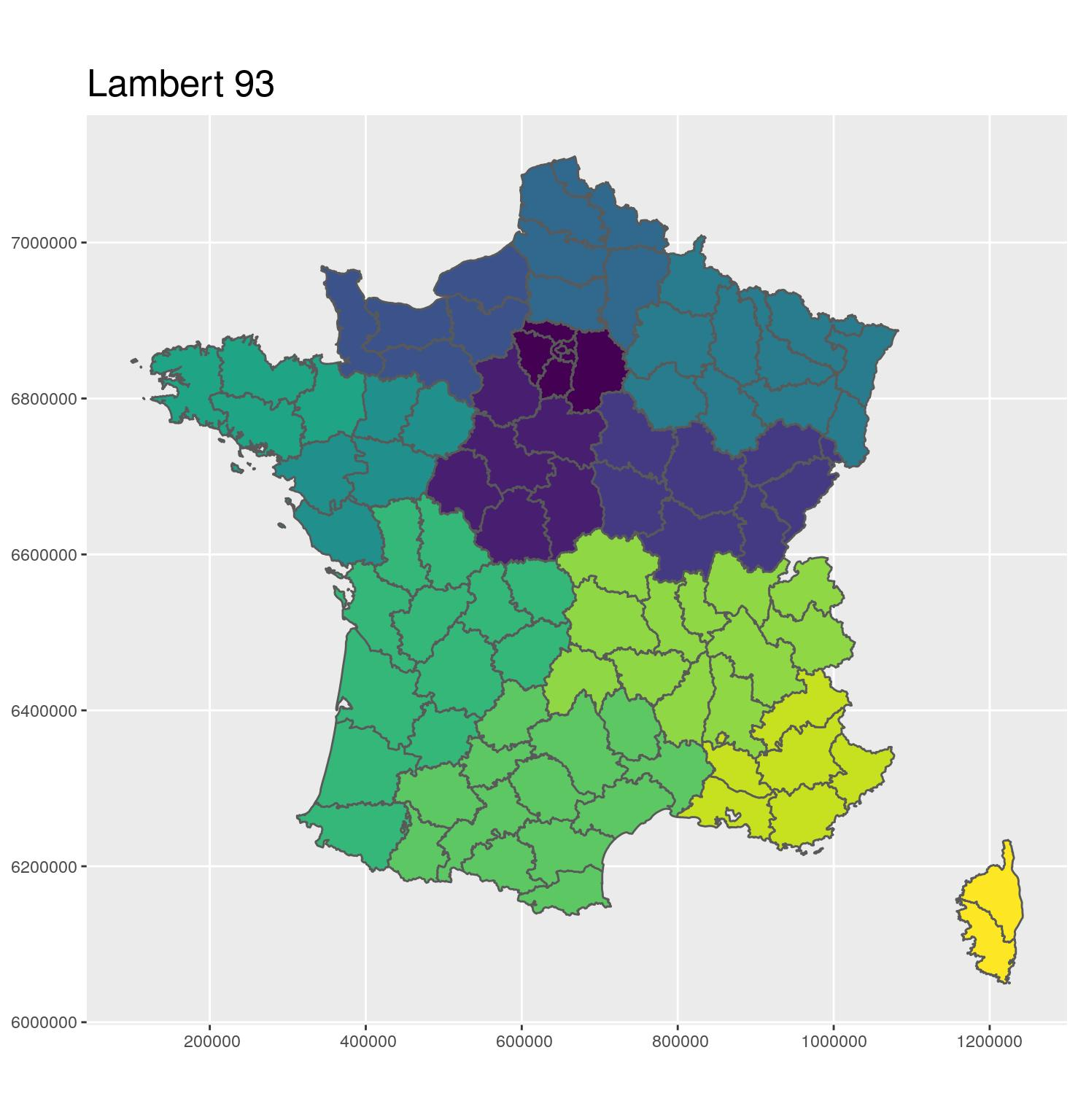A GIF to better see the difference

``````library(magick)
image_animate(c(oldlogo, newlogo), fps = 1, loop = 0)``````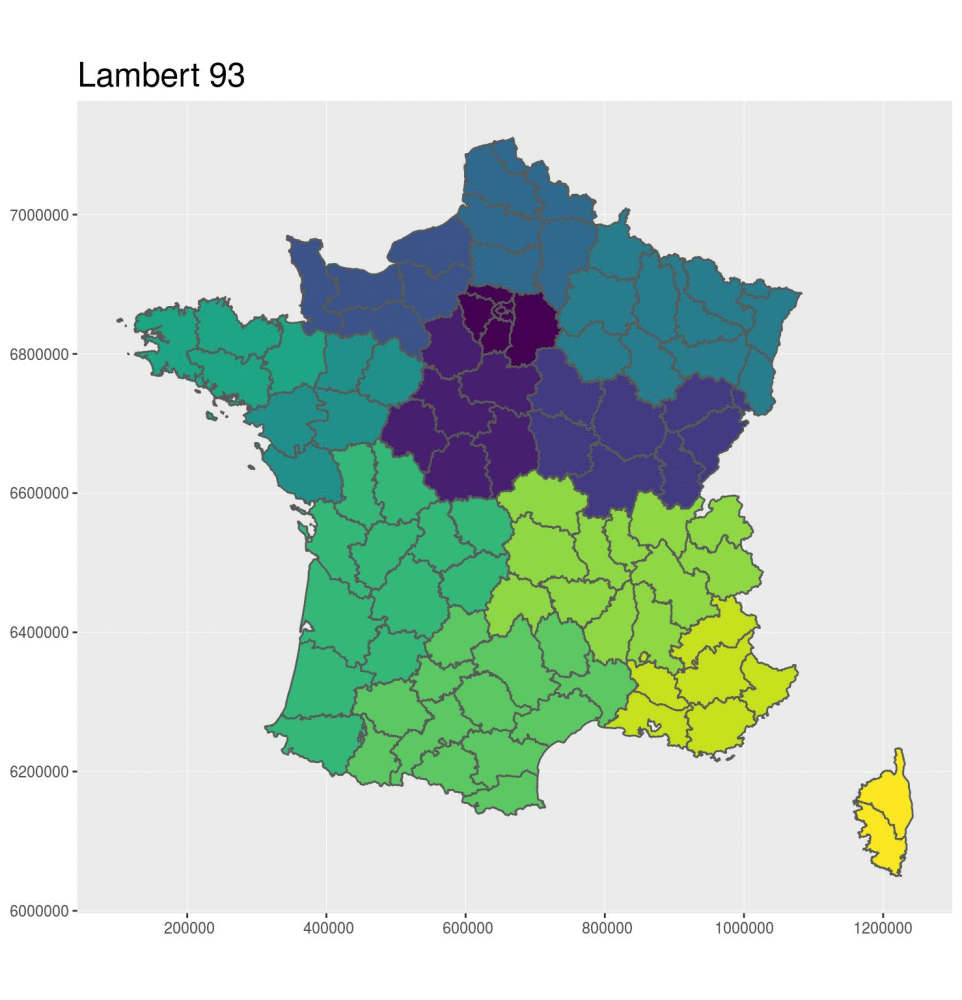### Spatial mapping and analysis with R

We will focus only on vector data. Raster data manipulation can be realized with the {raster} package, which is very powerful, but this is not the subject of this post.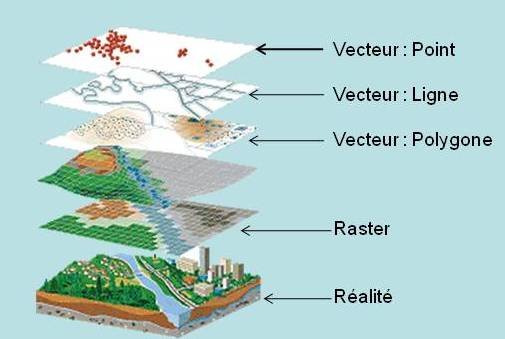### Vector layer file format

• With {sf} : Read with `st_read`.
• All formats managed by GDAL (http://www.gdal.org/)
• CSV, Mapinfo, Google Earth, GeoJSON, PostGIS, …
• ESRI™️ shapefile format
• Most commonly shared
• Attention: 4 files minimum (shp, shx, dbf, prj)

With {sf}, the files become classic dataframes.

``departements_L93[,2:3]``
``````## Simple feature collection with 96 features and 2 fields
## geometry type:  MULTIPOLYGON
## dimension:      XY
## bbox:           xmin: 99217.1 ymin: 6049646 xmax: 1242417 ymax: 7110480
## epsg (SRID):    2154
## proj4string:    +proj=lcc +lat_1=49 +lat_2=44 +lat_0=46.5 +lon_0=3 +x_0=700000 +y_0=6600000 +ellps=GRS80 +towgs84=0,0,0,0,0,0,0 +units=m +no_defs
## First 10 features:
##    CODE_DEPT       NOM_DEPT                       geometry
## 1         39           JURA MULTIPOLYGON (((886244.2 66...
## 2         42          LOIRE MULTIPOLYGON (((764370.3 65...
## 3         76 SEINE-MARITIME MULTIPOLYGON (((511688.8 69...
## 4         89          YONNE MULTIPOLYGON (((709449.1 67...
## 5         68      HAUT-RHIN MULTIPOLYGON (((992779.1 67...
## 6         28   EURE-ET-LOIR MULTIPOLYGON (((548948.9 68...
## 7         10           AUBE MULTIPOLYGON (((740396.5 68...
## 8         55          MEUSE MULTIPOLYGON (((846578.7 68...
## 9         61           ORNE MULTIPOLYGON (((425026.6 68...
## 10        67       BAS-RHIN MULTIPOLYGON (((998020.8 68...``````

Contrary to {sp} + {rgdal}

``str(as(departements_L93[,2:3], "Spatial"), max.level = 2)``
``````## Formal class 'SpatialPolygonsDataFrame' [package "sp"] with 5 slots
##   ..@ data       :'data.frame':  96 obs. of  2 variables:
##   ..@ polygons   :List of 96
##   ..@ plotOrder  : int [1:96] 57 12 27 69 76 21 63 18 65 26 ...
##   ..@ bbox       : num [1:2, 1:2] 99217 6049646 1242417 7110480
##   .. ..- attr(*, "dimnames")=List of 2
##   ..@ proj4string:Formal class 'CRS' [package "sp"] with 1 slot``````
``# str(as(departements_L93[,2:3], "Spatial"), max.level = 3)``

### Manipulate data with {dplyr}

• 🎉 Everything you know of {dplyr} works on {sf} spatial objetcs 🎉
• `%>%`
• `select`, `mutate` for attributes (= columns)
• `filter`, `arrange` for spatial features (= rows)

Example of manipulation

``````Bret_L93 <-
departements_L93 %>%
mutate_at(
vars(NOM_DEPT, NOM_REG),
tolower) %>%
select(CODE_DEPT, NOM_DEPT, NOM_REG) %>%
filter(NOM_REG == "bretagne")
Bret_L93``````
``````## Simple feature collection with 4 features and 3 fields
## geometry type:  MULTIPOLYGON
## dimension:      XY
## bbox:           xmin: 99217.1 ymin: 6704195 xmax: 400572.3 ymax: 6881964
## epsg (SRID):    2154
## proj4string:    +proj=lcc +lat_1=49 +lat_2=44 +lat_0=46.5 +lon_0=3 +x_0=700000 +y_0=6600000 +ellps=GRS80 +towgs84=0,0,0,0,0,0,0 +units=m +no_defs
##   CODE_DEPT        NOM_DEPT  NOM_REG                       geometry
## 1        35 ille-et-vilaine bretagne MULTIPOLYGON (((330478.6 68...
## 2        22   cotes-d'armor bretagne MULTIPOLYGON (((259840 6876...
## 3        29       finistere bretagne MULTIPOLYGON (((116158.5 67...
## 4        56        morbihan bretagne MULTIPOLYGON (((256230.1 67...``````
``````ggplot(Bret_L93) +
geom_sf(aes(fill = NOM_DEPT)) +
scale_fill_viridis_d()``````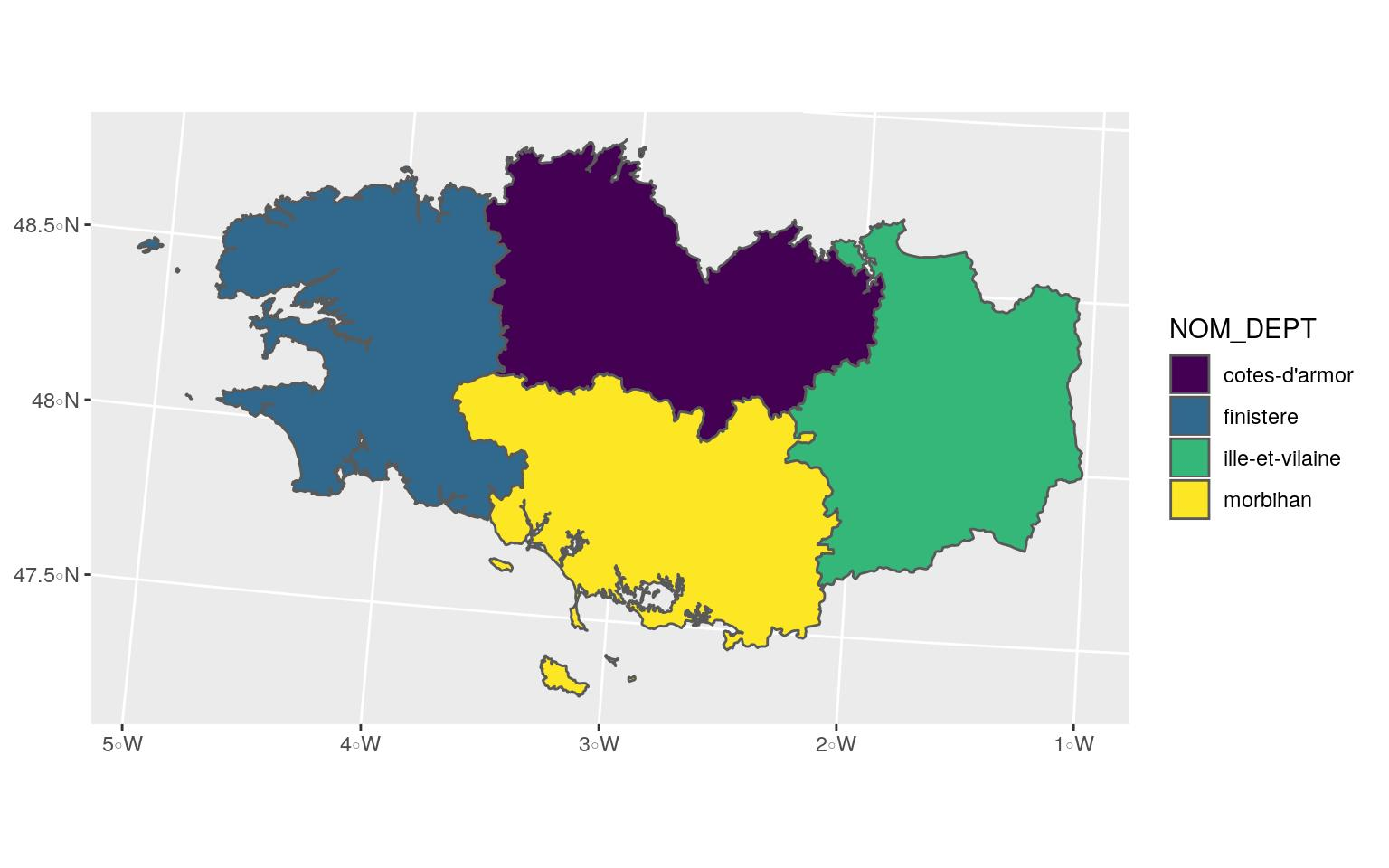• Merge spatial features with `group_by` + `summarize`

Before

``````g_dept +
ggtitle("Départements")``````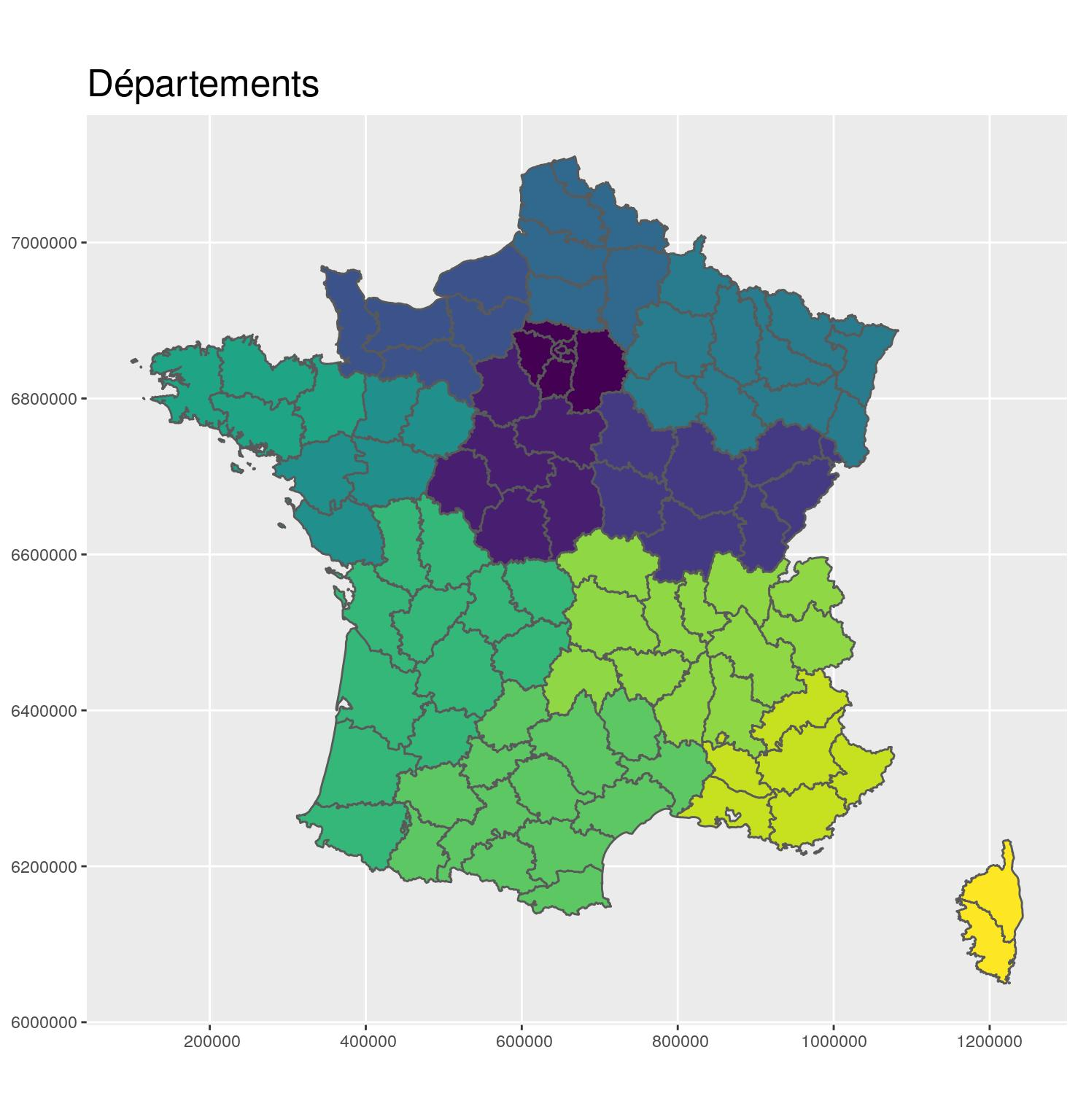Merge

``````region_L93 <- departements_L93 %>%
group_by(CODE_REG, NOM_REG) %>%
summarize()``````

After

``````g_region <- ggplot(region_L93) +
aes(fill = CODE_REG) +
scale_fill_viridis_d() +
geom_sf() +
coord_sf(crs = 2154, datum = sf::st_crs(2154)) +
guides(fill = FALSE) +
ggtitle("Régions") +
theme(title = element_text(size = 16))
g_region``````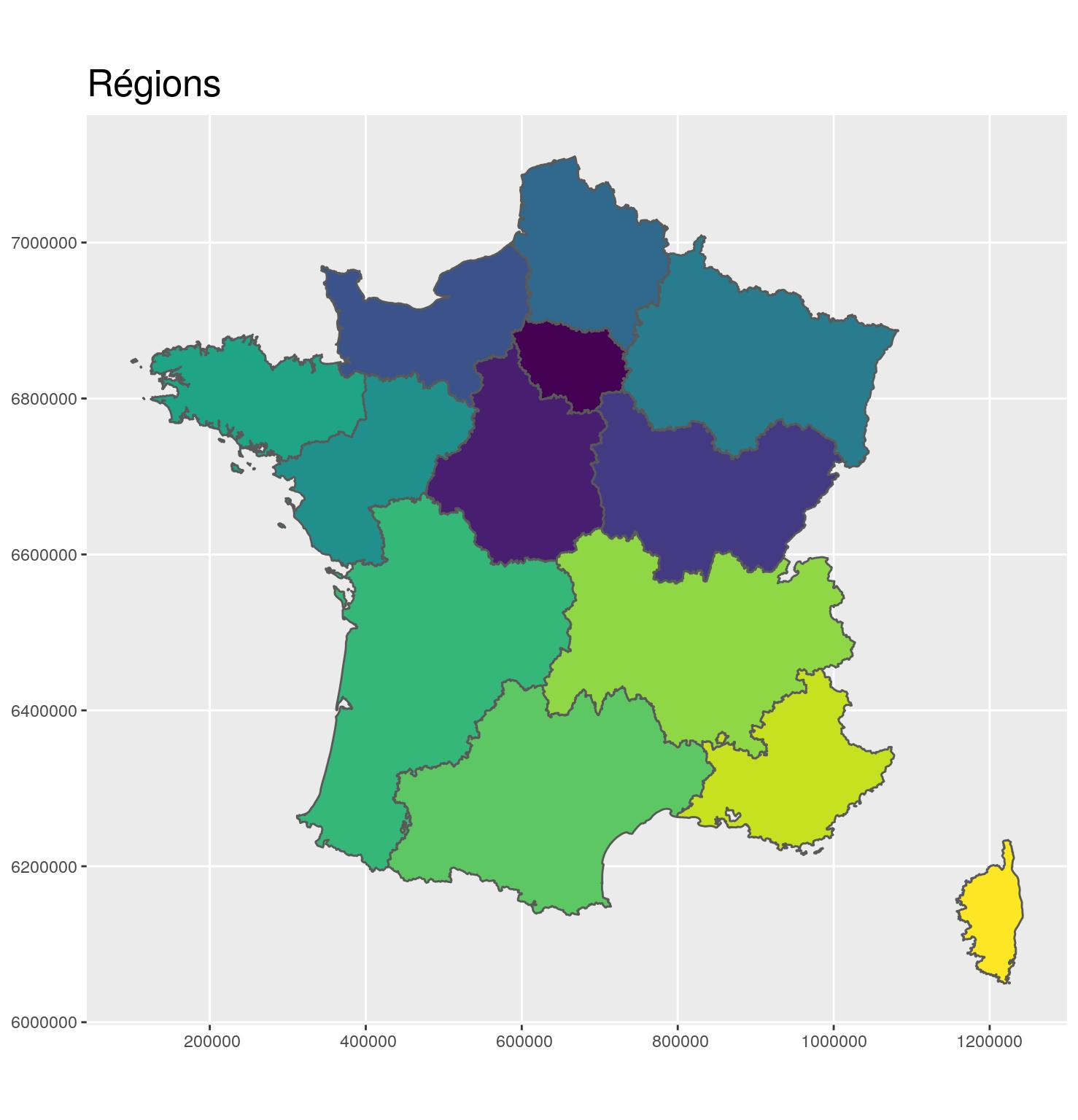• Classical data join between a shapefile of French communes and a CSV file of French communes having a maternity with `left_join`.

``````# extraWD <- "."
# -- Communes --
if (!file.exists(file.path(extraWD, "communes.zip"))) {
githubURL <- "https://github.com/statnmap/blog_tips/raw/master/2018-07-14-introduction-to-mapping-with-sf-and-co/data/communes.zip"
}
unzip(file.path(extraWD, "communes.zip"), exdir = extraWD)

# -- Maternites --
if (!file.exists(file.path(extraWD, "communes.zip"))) {
githubURL <- "https://github.com/statnmap/blog_tips/raw/master/2018-07-14-introduction-to-mapping-with-sf-and-co/data-mater/Maternite_2004-2016.csv"
}

# Read shapefile of French communes
communes <- st_read(dsn = extraWD, layer = 'COMMUNE', quiet = TRUE) %>%
select(NOM_COM, INSEE_COM)
# Read file of maternities for 2016
filter(an == 2016)``````

Jointure

``````# Join database with shapefile by attributes
maternites_L93 <- communes %>%
right_join(data.maternite,
by = "INSEE_COM") %>%
st_transform(2154) ``````
``````g_region +
geom_sf(data = maternites_L93, fill = "white", colour = "white") +
coord_sf(crs = 2154, datum = sf::st_crs(2154)) +
ggtitle("Communes avec une maternité en 2016")``````### Spatial join

Join layers with `st_intersection` to spatially select and retrieve information from another spatial layer based on geographic positions.

• Points, polygons, lines with polygons

Spatial join

``````maternites_Bret_L93 <- maternites_L93 %>%
st_intersection(Bret_L93)``````
``## Attributs du fichier 'Maternités' avant intersection``
``````##  "NOM_COM"     "INSEE_COM"   "an"          "Code_Postal" "n"
##  "geometry"``````
``## Attributs après Intersection avec région``
``````##  "NOM_COM"     "INSEE_COM"   "an"          "Code_Postal" "n"
##  "CODE_DEPT"   "NOM_DEPT"    "NOM_REG"     "geometry"``````
``````ggplot() +
geom_sf(data = departements_L93, colour = "grey20") +
geom_sf(data = Bret_L93, fill = "#440154", colour = "grey60") +
geom_sf(data = maternites_Bret_L93,
fill = "#FDE725", colour = "#FDE725") +
coord_sf(crs = 2154, datum = sf::st_crs(2154),
xlim = st_bbox(Bret_L93)[c(1,3)],
ylim = st_bbox(Bret_L93)[c(2,4)]
) +
theme(title = element_text(size = 16),
panel.background = element_rect(fill = "#66BDFF")) +
ggtitle("Communes bretonnes avec maternité")``````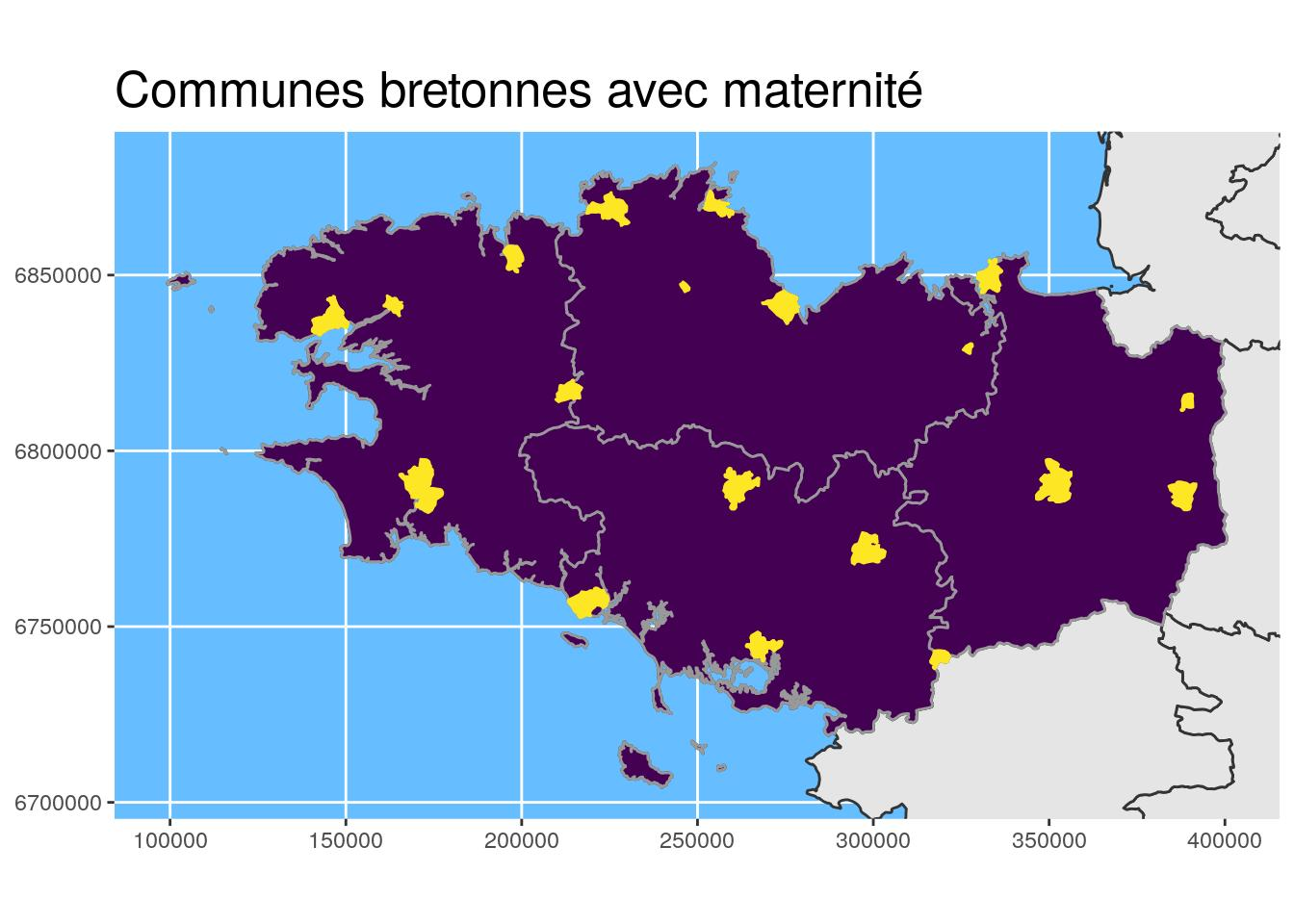### Geomatics

• Buffer area calculation to highlight the Brittany region with `st_buffer`

Buffer area

``````# Buffer area for Brittany
Bret_buffer10_L93 <-
Bret_L93 %>%
st_buffer(
dist =
units::set_units(10, km)
) %>%
st_cast() # useful sometimes !

# Buffer Bretagne for larger study area bbox only
Bret_buffer30_L93 <-
Bret_L93 %>%
st_buffer(
dist =
units::set_units(30, km)
) ``````
``````ggplot() +
geom_sf(data = departements_L93, colour = "grey50",
fill = "white") +
geom_sf(data = st_union(Bret_buffer10_L93), fill = "#FDE725", #"#440154",
colour = "grey80", size = 1.1) +
geom_sf(data = st_union(departements_L93), colour = "grey20",
fill = NA, size = 1.2) +
geom_sf(data = Bret_L93, fill = "#440154", colour = "grey60") +
# scale_fill_manual(values = c("#f15522", "#15b7d6", "#ada9ae")) +
coord_sf(crs = 2154, datum = sf::st_crs(2154),
xlim = st_bbox(Bret_buffer30_L93)[c(1,3)],
ylim = st_bbox(Bret_buffer30_L93)[c(2,4)]
) +
theme(title = element_text(size = 16),
panel.background = element_rect(fill = "#66BDFF")) +
ggtitle("Zone tampon autour de la Bretagne")``````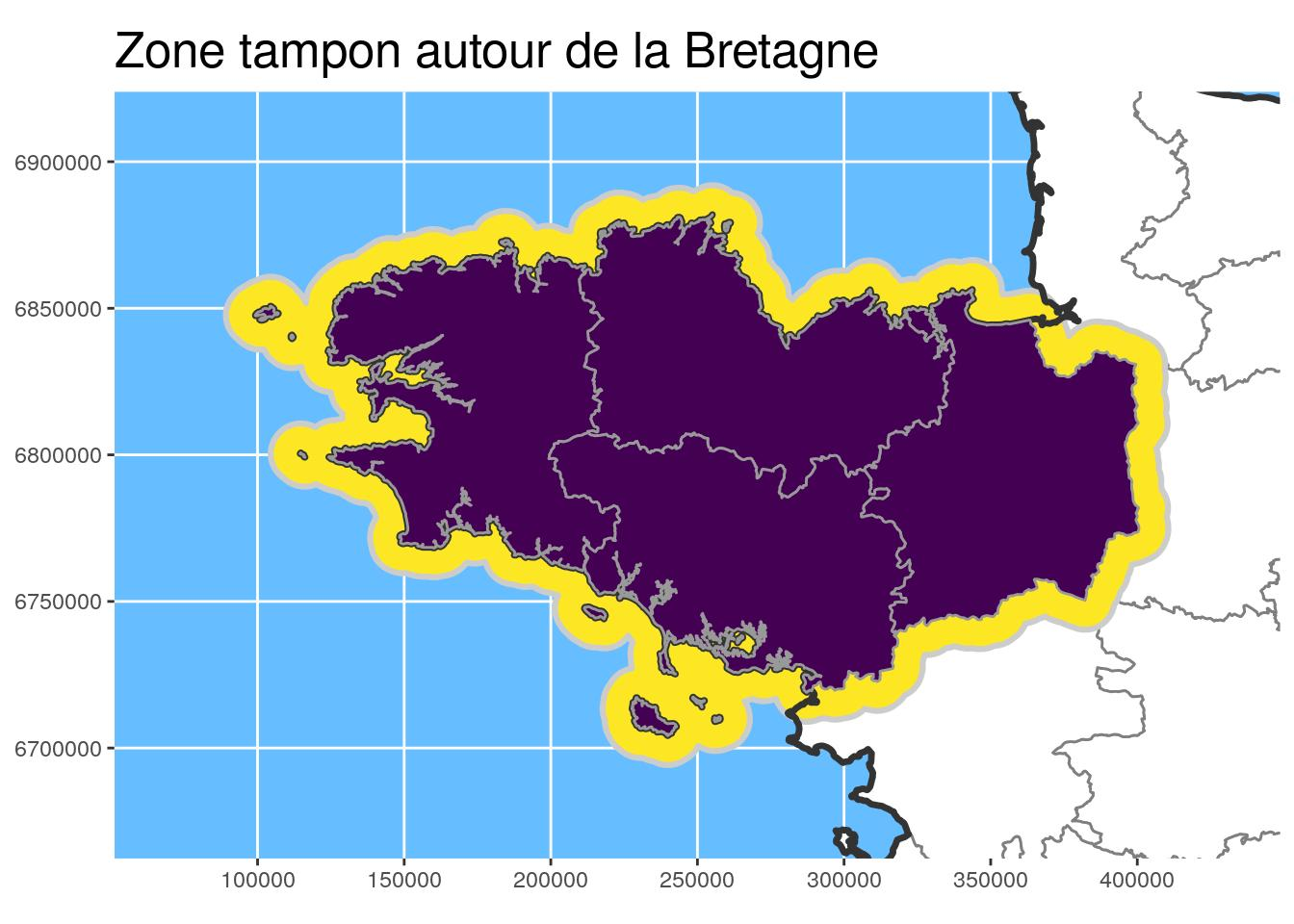By coupling buffer areas with other utility functions, we can draw distance zones as the stork flies.

• Retrieve polygon centroids of communes in Brittany with a maternity with `st_centroid`.
• Unions with `st_union`
• Difference with `st_difference`

In the code chunk below, the `dist_circles` function allows to create these buffer areas around a point or any other {sf} object.

Function

``````# Define couples distances - years to buffer
#' @param x sf object
#' @param dists  c(10000, 25000, 50000, 75000)
#' @param ans  unique(maternites_pt\$an)

dist_circles <- function(x,
dists = units::set_units(c(10, 25, 50), km),
ans,
x.limits) {
# browser()
if (!missing(ans)) {
dists_ans <- data.frame(
dists = rep(dists, length(ans)),
ans = rep(ans, each = length(dists))
)
# Create buffer areas for each distances / year
pts_buf <- purrr::map2(
dists_ans\$dists, dists_ans\$ans,
~st_buffer(
filter(x, an == .y),
.x) %>%
mutate(
dist = .x,
)
) %>%
do.call("rbind", .) %>%
st_cast() %>%
mutate(dist.leg = glue::glue("<{dist/1000} km"))

# Define triplet big/small-distance/year
big_small <- data.frame(
big_dist = dists[rep(2:length(dists), length(ans))],
small_dist = dists[rep(1:(length(dists) - 1), length(ans))],
an = ans[rep(1:length(ans), each = length(dists) - 1)]
)
# Remove part of polygons overlapping smaller buffer
pts_holes <- big_small %>%
split(1:nrow(big_small)) %>%
purrr::map(
~st_difference(
filter(pts_buf, dist == .\$big_dist, an == .\$an),
filter(pts_buf, dist == .\$small_dist, an == .\$an)
)
) %>%
do.call("rbind", .) %>%
select(-contains(".1")) %>%
st_cast()

# Add smallest polygons and re-order distance names for legend
pts_holes_tot <- pts_holes %>%
rbind(
filter(pts_buf, dist == min(dists))
) %>%
arrange(an, dist) %>%
mutate(dist = forcats::fct_reorder(dist.leg, dist))

} else {
pts_buf <- purrr::map(
dists,
~st_buffer(x, .x) %>%
mutate(dist = .x)
) %>%
do.call("rbind", .) %>%
st_cast() %>%
mutate(dist.leg = as.character(dist))

# Define triplet big/small-distance/year
dists_order_char <- sort(dists) %>% as.character()
big_small <- data.frame(
big_dist = tail(dists_order_char, -1),
)

# Remove part of polygons overlapping smaller buffer
pts_holes <- big_small %>%
split(1:nrow(big_small)) %>%
purrr::map(
~st_difference(
filter(pts_buf, dist.leg == .\$big_dist),
filter(pts_buf, dist.leg == .\$small_dist) %>%
select(geometry) %>% st_union()
)
) %>%
do.call("rbind", .) %>%
select(-contains(".1")) %>%
st_cast()

# Add smallest polygons and re-order distance names for legend
pts_holes_tot <- pts_holes %>%
rbind(
filter(pts_buf, dist.leg == dists_order_char)
) %>%
arrange(dist) %>%
mutate(dist = forcats::fct_reorder(dist.leg, dist))

}

pts_holes_fr <- st_intersection(pts_holes_tot,
dplyr::select(x.limits, geometry))

return(pts_holes_fr)

}``````

Calculation of distance circles

``````# Centroides des communes des maternités
maternites_centroid_Bret_L93 <-
maternites_Bret_L93 %>%
st_centroid()

# Circles around centroids
dists <- c(5, 15, 25, 50)
maternites_circles_L93 <- dist_circles(
maternites_centroid_Bret_L93,
dists = units::set_units(dists, km),
x.limits = Bret_L93)``````

Figure

``````# Plot and define color according to dist
ggplot() +
geom_sf(data = departements_L93, colour = "grey50",
fill = "white") +
geom_sf(data = st_union(Bret_buffer10_L93), fill = "#FDE725", #"#440154",
colour = "grey80", size = 1.1) +
geom_sf(data = maternites_circles_L93,
aes(fill = dist),
colour = NA) +
scale_fill_brewer(direction = -1) +
geom_sf(data = Bret_L93, fill = NA, colour = "grey60") +
geom_sf(data = st_union(departements_L93), colour = "grey20",
fill = NA, size = 1.2) +
# scale_fill_manual(values = c("#f15522", "#15b7d6", "#ada9ae")) +
coord_sf(crs = 2154, datum = sf::st_crs(2154),
xlim = st_bbox(Bret_buffer30_L93)[c(1,3)],
ylim = st_bbox(Bret_buffer30_L93)[c(2,4)]
) +
theme(title = element_text(size = 16),
panel.background = element_rect(fill = "#66BDFF")) +
ggtitle("Distances à vol d'oiseau du centre\nd'une commune avec maternité")``````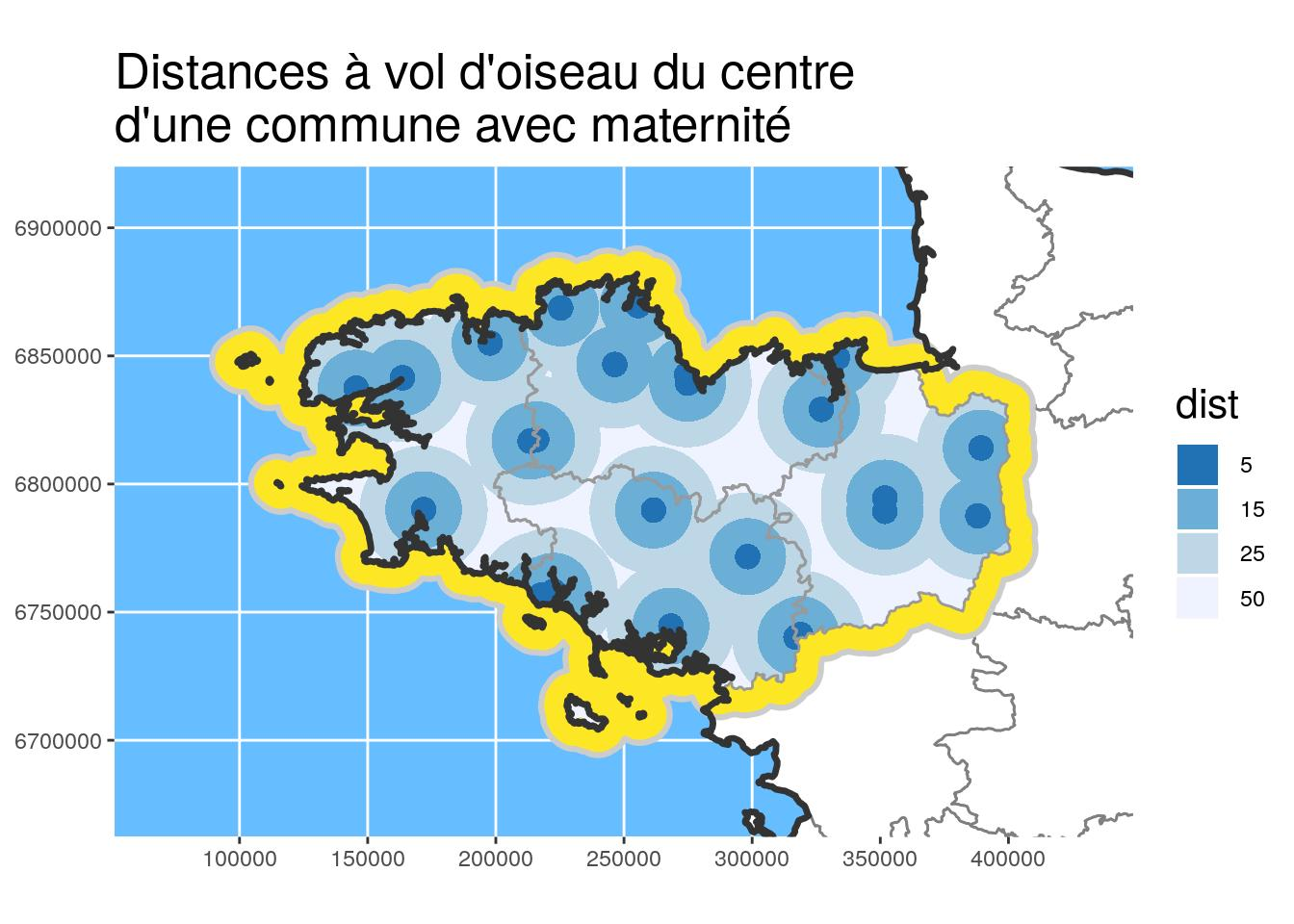I used a similar technique in my previous article “Polygons tint bands with leaflet and package {sf}”

## Draw maps on R

non-exhaustive personal list of the tools I use the most.

### Maps with {ggplot2}

You may have noticed that the previous maps were all made with {ggplot2}. It is now possible with the last version of {ggplot2} (v3.0+) recently released on CRAN ! I also put {viridis} colours in the previous maps because it is also possible in a simple way with `scale_fill_viridis_*` in the new version of {ggplot2}. If you want to know more about {viridis}, have a look at Viridis ThinkR blog post.

• `geom_sf`: recognizes geometry
• `coord_sf`: axis limits and CRS
• Several layers can be added by specifying `geom_sf(data = ...)`
``````ggplot() +
geom_sf(data = departements_L93, colour = "grey40",
fill = "white", size = 1.1) +
geom_sf(data = maternites_circles_L93,
aes(fill = dist), colour = NA) +
scale_fill_brewer(direction = -1) +
coord_sf(crs = 2154, datum = sf::st_crs(2154),
xlim = st_bbox(Bret_buffer30_L93)[c(1,3)],
ylim = st_bbox(Bret_buffer30_L93)[c(2,4)]
) +
theme(title = element_text(size = 16),
panel.background = element_rect(fill = "#66BDFF")) +
ggtitle("Distances à vol d'oiseau du centre\nd'une commune avec maternité")``````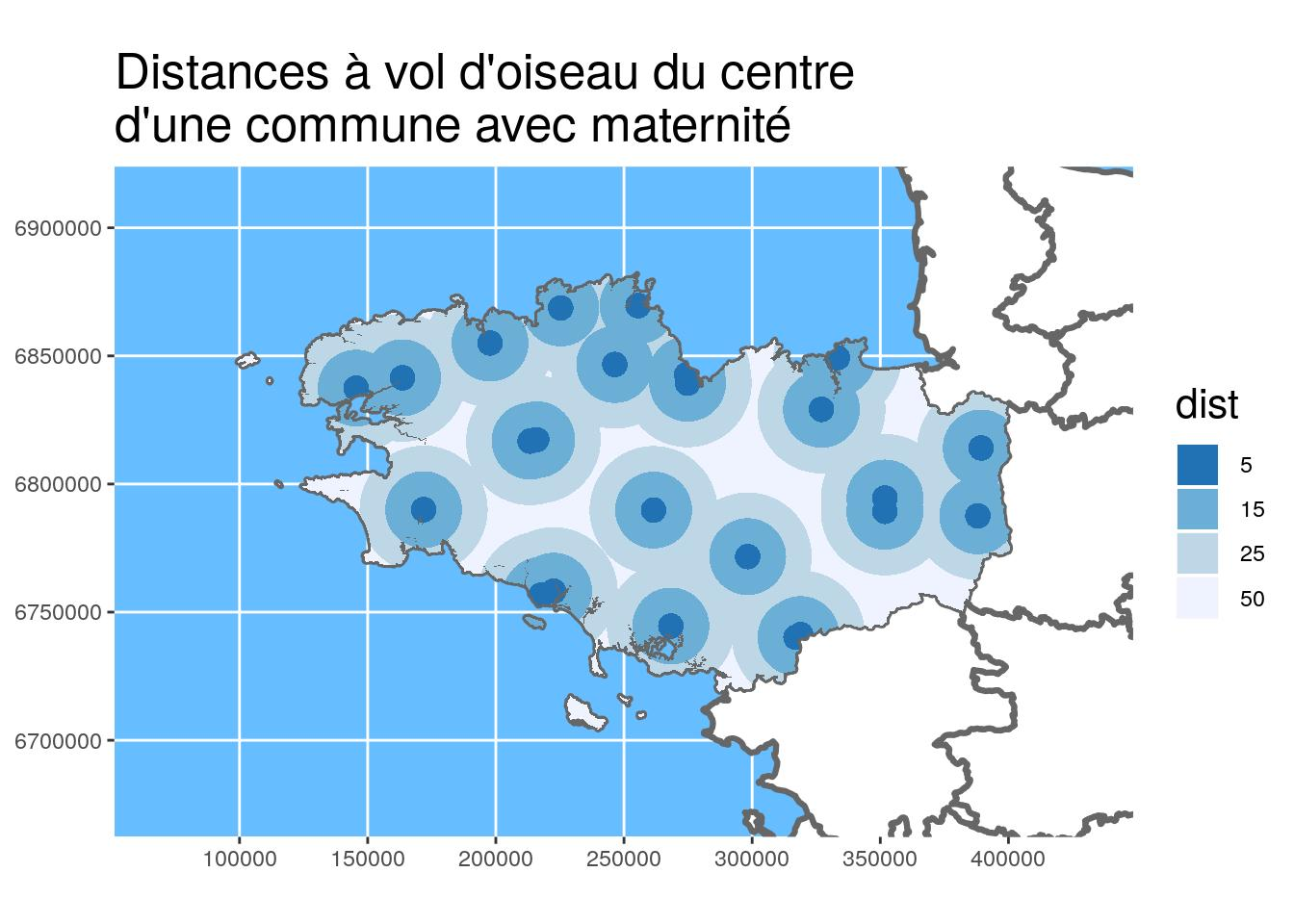### Maps with {tmap}

I like {tmap} because it was created to draw maps. Different spatial data formats are supported ({sp}, {sf}, {raster}), North scale and arrow are managed natively. You can add multiple layers on top of each other using `tm_shape`.

Some functions :

• `tm_shape`
• `tm_dots` : points
• `tm_fill` : polygons
• `tm_text` : text
• `tm_scale_bar`
• `tm_compass`
``````# Load background map
# data(Europe) # deprecated in tmap 2.0
data(World)
Europe <- World %>%
filter(continent == "Europe" & name != "France")

# Map
tm <- tm_shape(Europe) +
tm_polygons() +
tm_shape(departements_L93, is.master = TRUE) +
tm_fill(col = "NOM_DEPT", legend.show = FALSE, palette = "Set1") +
tm_borders("grey30") +
tm_shape(maternites_circles_L93) +
tm_fill(col = "dist", palette = "-Blues") +
tm_scale_bar(position = c("right", "bottom"), size = 1) +
tm_compass(position = c("right", "top"), size = 1.8) +
# tm_style_natural() #deprecated in tmap 2.0
tm_style("natural")``````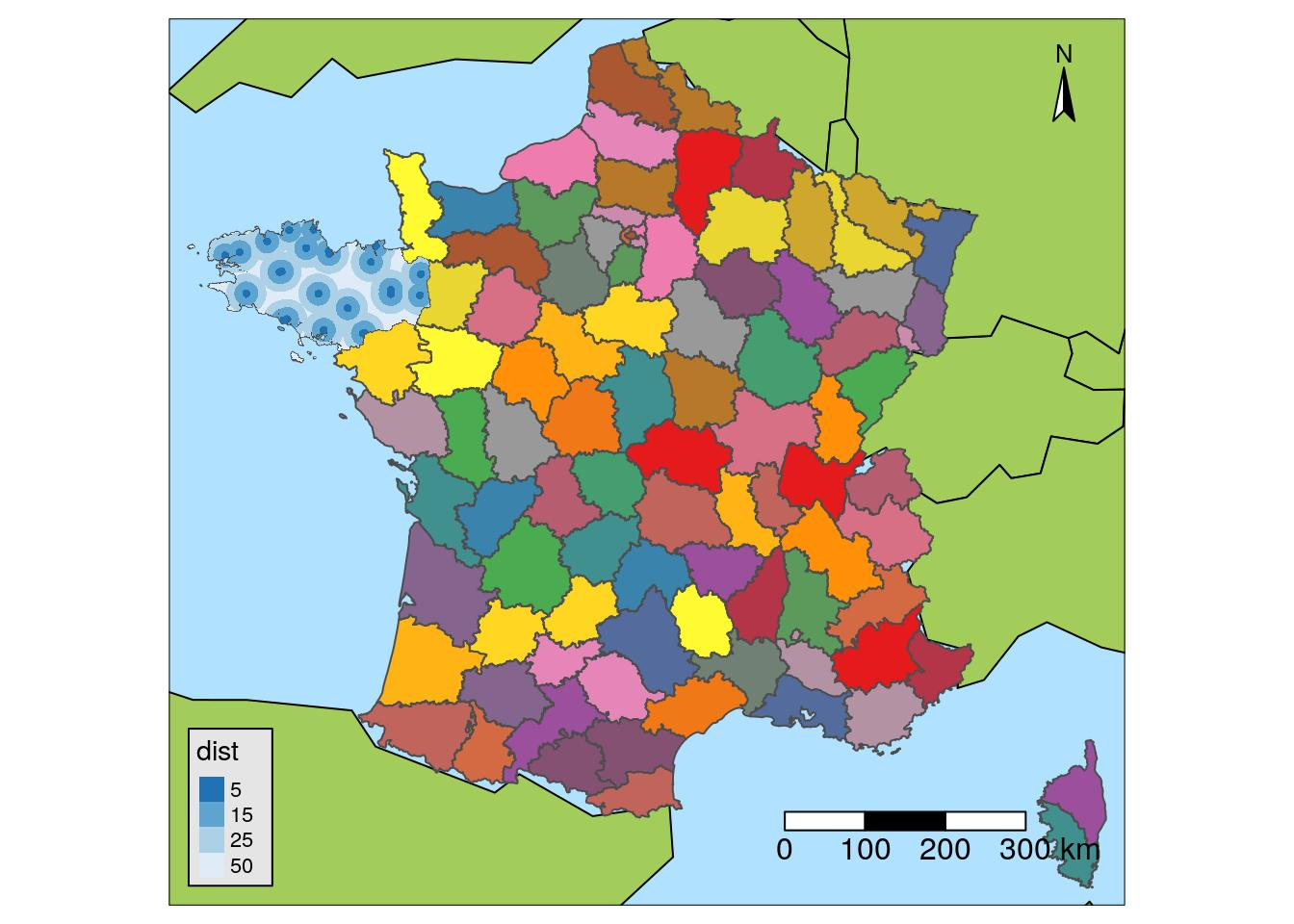### Interactive maps with {leaflet}

To provide interactive maps, you can use {leaflet}:

• Accept {sf} objects
• CRS: 4326
• Create color palettes with `colorFactor` for example
• `addTiles()` : Maps in background
• `addMarkers` : Points
• `addPolygons` : Polygons
``````departements_wgs84 <-
st_transform(departements_L93, crs = 4326)

factpal <- colorFactor(topo.colors(5), departements_wgs84\$NOM_REG)

m <- leaflet() %>%